• 当前不少网友表示自己才接触Matlab这款应用，还不晓得怎么定义变量的...符号变量定义方法例如: sym x 或 syms x ，两者有区别也有共同点。情况二：计算过程当中的存储数值的变量可以不必定义，随时需要，随时定义，...
当前不少网友表示自己才接触Matlab这款应用，还不晓得怎么定义变量的操作，接下来就为你们分享Matlab定义变量的操作步骤，大家都来一起学习吧。Matlab定义变量的操作步骤情况一：符号变量一定要定义，定义之后才能使用。如下面的例子，未定义就会出错。符号变量定义方法例如: sym x 或 syms x ，两者有区别也有共同点。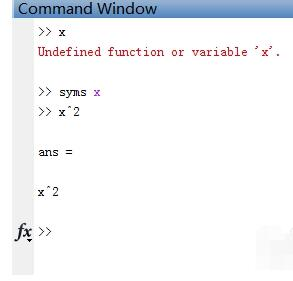情况二：计算过程当中的存储数值的变量可以不必定义，随时需要，随时定义，但有时若变量很多，那最好提前声明，声明时，可直接赋予0值，并且注释，这样方便以后区分，避免混淆。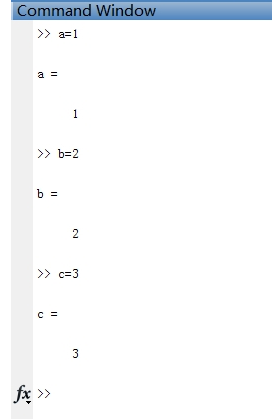情况三：矩阵和向量对于矩阵一般都是需定义的，定义好矩阵之后，才方便访问/修改矩阵的指定元素，矩阵声明(定义)的方法，常用的 就是声明为零矩阵zeros(M,N),或者单位矩阵ones(M,N),eye(M,N)等。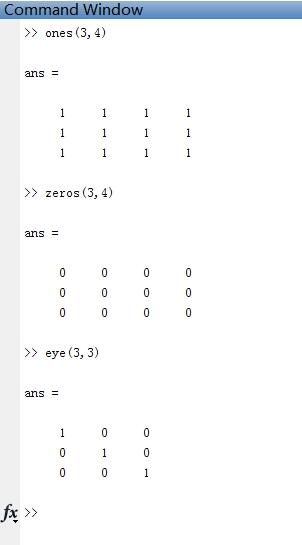Matlab相关攻略推荐:上面就是小编为大家带来的Matlab定义变量的操作方法，一起来学习学习吧。相信是可以帮助到一些新用户的。
展开全文• 全局变量定义与使用 注意：全局变量的定义和使用，都要使用global xxx 命令。 %在主程序中定义全局变量 global TR_ANTENNA %定义全局变量 global VALUE_SNR %这两个全局变量被用来存储循环变量，如 for tr = ...
问题描述

当在循环中调用自定义函数时，函数中的某些参数需要随着循环的执行而更新。如何能在自定义的函数内部使用这些不断更新的参数呢？全局变量可以解决这一问题。

全局变量定义与使用

注意：全局变量的定义和使用，都要使用 global xxx 命令。

%在主程序中定义全局变量

global TR_ANTENNA  %定义全局变量
global VALUE_SNR

%这两个全局变量被用来存储循环变量，如

for tr = 1:M

TR_ANTENNA = tr;

...

for i = 1:length(SNR)

VALUE_SNR = 10^(SNR(i)/10);

...

========================================================================
%在自定义函数 fitnessfcn() 里面要用到这两个全局变量，因为函数调用命令是在内循环里，所以需要全局变量来传递参数，如

function y = fitnessfcn(x)

global TR_ANTENNA   %使用全局变量
global VALUE_SNR

...

P_1_ga = (gammainc(m(1)*eta_1_ga/Omega(1)/VALUE_SNR,m(1))/gamma(m(1)))^TR_ANTENNA;


 https://ww2.mathworks.cn/help/matlab/ref/global.html

 https://blog.csdn.net/lansatiankongxxc/article/details/23767105

展开全文• % 得到的结果是 x=1 在matlab中，自己定义变量优先级高于本身的优先集，所以得到的结果是1 %所以在定义的时候一定要注意不要使用内部函名称来定义变量，这个和C语言有所区别 %matlab是先在当前文件夹中搜索,然后是...
慕课matlab学习
第一章-01

% matlab 基本操作

x=sin(1); %调用的是 sin（x)数学函数
sin=[1,2,3,4,5]; %调用的是 sin[]的一个数组
x=sin(1);% 得到的结果是 x=1  在matlab中，自己定义的变量优先级高于本身的优先集，所以得到的结果是1
%所以在定义的时候一定要注意不要使用内部函名称来定义变量，这个和C语言有所区别
%matlab是先在当前文件夹中搜索,然后是在其搜索路径搜索
%也就是说，当出现连个名字一样的 .m文件时，需要先运行的是当前文件夹的。m文件




展开全文• 定义变量a，b，c，计算输出d >> a=-3;b=2;c=5;>> d=(a^2+b)/c;>> d=(a^2+b)/c d = 2.2000 系统默认变量 ans 创建一个.m文件 在主界面中edit+ 执行时直接在命令窗口写文件名字 定义...
 定义变量a，b，c，计算输出d
>> a=-3;b=2;c=5;>> d=(a^2+b)/c;>> d=(a^2+b)/c
d =
2.2000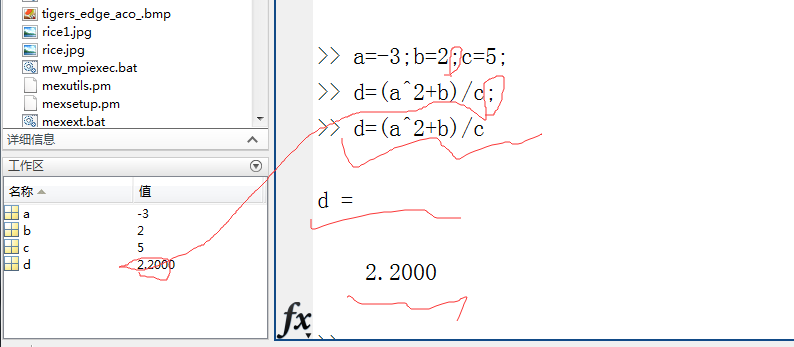系统默认变量  ans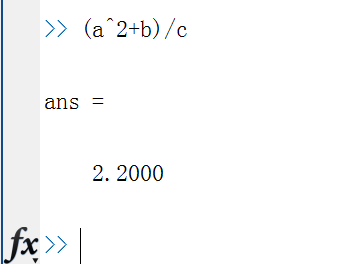创建一个.m文件
在主界面中edit+
执行时直接在命令窗口写文件名字
定义函数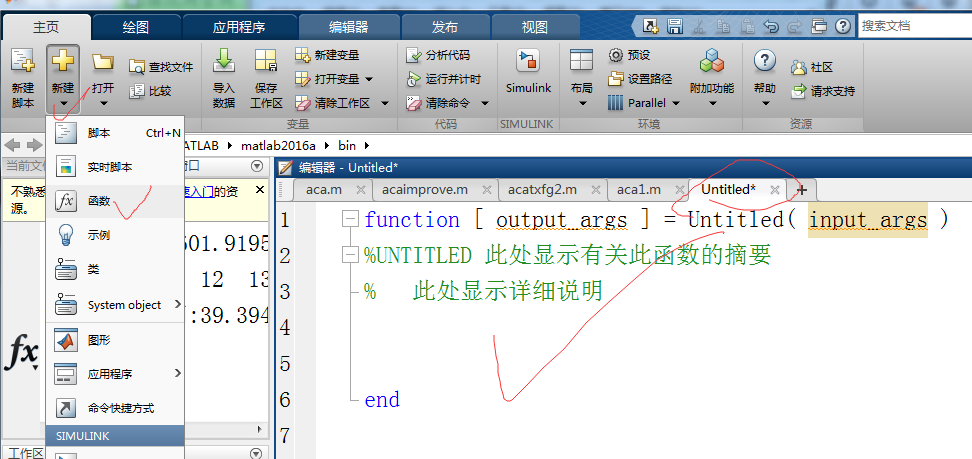保存函数，名字要一致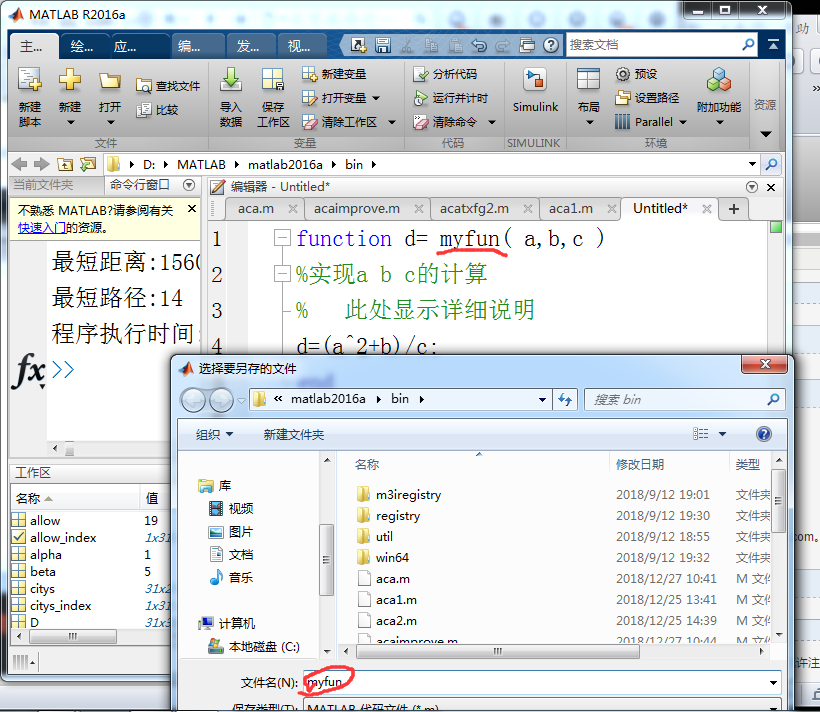但是函数只能被调用，不能直接运行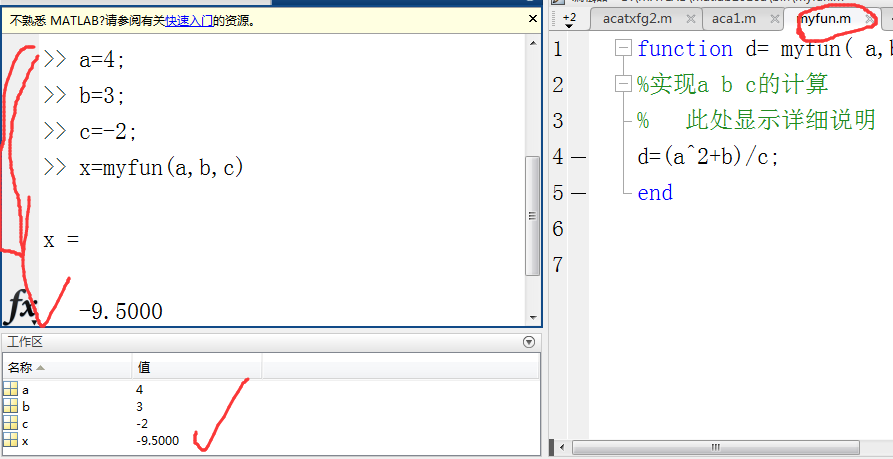或者直接赋值
>> x=myfun(3,2,5)
x =
2.2000
转载于:https://www.cnblogs.com/fanglijiao/p/10257617.html
展开全文• ## matlab变量

千次阅读 2013-07-29 21:55:02
MATLAB中的变量命名规则 1.变量名必须以字符开头，后面可以跟字母、数字、下划线，但不能用空格和标点符号（这个跟C标准相同）；...局部变量：函数体内都有自己定义变量，不能从其它函数和MATLAB工作
• 第二章 Matlab变量Matlab的特殊常量变量的命名规则变量的定义与赋值变量的显示变量的存取变量的清除变量的检查 Matlab的特殊常量 变量的命名规则 变量的定义与赋值 变量的显示 变量的存取 变量的清除 变量的...
• matlab变量，关键字 MATLAB变量命名规则： 变量名区分大小写。 变量名长度不得超过 31 位，第 31 个字符之后的字符将被 MATLAB 语言所忽略。 变量名以字母开头，可以是字母、数字、下划线组成，但不能使用标点。 &...
• matlab定义全局变量并赋值，在子函数中再次定义全局变量。 global x x=10; %子函数 function y= fct() global x; end
• popsize=10 for i=1:popsize if i==1 ... #循环定义order1~order10等变量 end end for o=1:3 d=strcat('moudle_',num2str(o)) end %最后得到的输出为： 'moudle_1', 'moudle_2', 'moudle_3' ...
• Matlab 变量及命名规则 Matlab 中所有的变量都是用矩阵形式来表示的 即所有的变量都表示一个矩 阵或者一个向量其命名...Matlab 系统预定义变量 表 3-2 Matlab 中预定义变量 ans 预设的计算结果的变量Matlab
• ## 8.1 MATLAB变量

千次阅读 2016-11-30 19:04:14
在程序中会经常定义一些变量来保存和处理数据，从本质上看，变量代表了一段可操作的内存，也可以认为变量是...在MATLAB中，变量不需要预先声明就可以进行赋值。变量的命名遵循以下规则： （1）变量名和函数名对字母的
• Matlab中，变量名是以字母开头，后接字母、数字或下划线的的字符序列，最多63个字符。 matlab变量名区分大小写 标准函数名以及命令名必须用小写字母 赋值语句两种形式 变量=表达式 表达式 预定义变量 它是系统...
• 1.2MATLAB数值数据l 数值数据类型的分类l 数值数据的输出格式l 常用数学函数内部函数1．数值数据类型的分类l 整型l 浮点型l 复数型(1)整型1．数值数据类型的分类无符号整数：无符号8位整数、无符号16位整数、无符号...
• c=10^(-3)/2/pi/650; t1=0; t2=0.034/c; syms x; Q=double(int(1/c*sqrt(x*x+c*c),0.022,0.056)); S=double(int(sqrt((0.022+c*x)^2+c*c),t1,t2)); Q/1.3/60 S/1.3/60 w=1.3/sqrt(0.05*0.05+c*...alph=c*w^4*0.05/1....
• 第二节 MATLAB变量 ——MATLAB语言基础 1.MATLAB的特殊常量 MATLAB中的特殊常量指的是一些预先定义好的数值变量，如下表所示： 含义 符号 圆周率π pi 机器的...编程语言
• MATLAB定义变量小细节（1） linspace（a,b,n）表示在（a,b）区间中等距的取n个点； x=a:n:b 表示在（a,b）区间中以n的距离取点
• 变量 含义 ... MATLAB 定义的正的极小值 / 浮点数的相对精确度 Inf / inf 无穷大 NaN 无法定义一个数 pi 圆周率π flops 浮点运算次数 i / j 虚数单位−1−−−√ realmax ...
• Matlab 量及命名 Matlab 中所有的 量都是用矩 形式来表示的 即所有的 量都表示一个矩 或者一个向量其...点 Matlab 系 定 的 量 表 3-2 Matlab 中预定义变量 ans 预设的计算结果的变量名 eps Matlab 定义的正的极小
• 使用 detectImportOptions 创建导入选项，设置多个变量的数据类型，然后使用 readtable 读取数据。创建一个选项对象。opts = detectImportOptions('patients.xls');检查变量的当前(检测到的)数据类型。disp([opts....
• Matlab变量及命名规则 Matlab中所有的变量都是用矩阵形式来表示的，即所有的变量都表示一个矩阵或者一个向量。其命名规则如下： （1）变量名对大小写敏感； （2）变量名的第一个字符必须为英文字母，其长度不能...
• 需要在“用到该全局变量”每个function函数里都进行声明： 即可完成
• 在C/C++里可以用宏定义或者全局常量，matlab也有方法实现。 1. oop matlab R2008a开始支持面向对象了。在类定义中可以定义些类常属性，就是可以定义常量，如 classdef MyConstants properties (Constant = ......

# matlab变量定义matlab 订阅